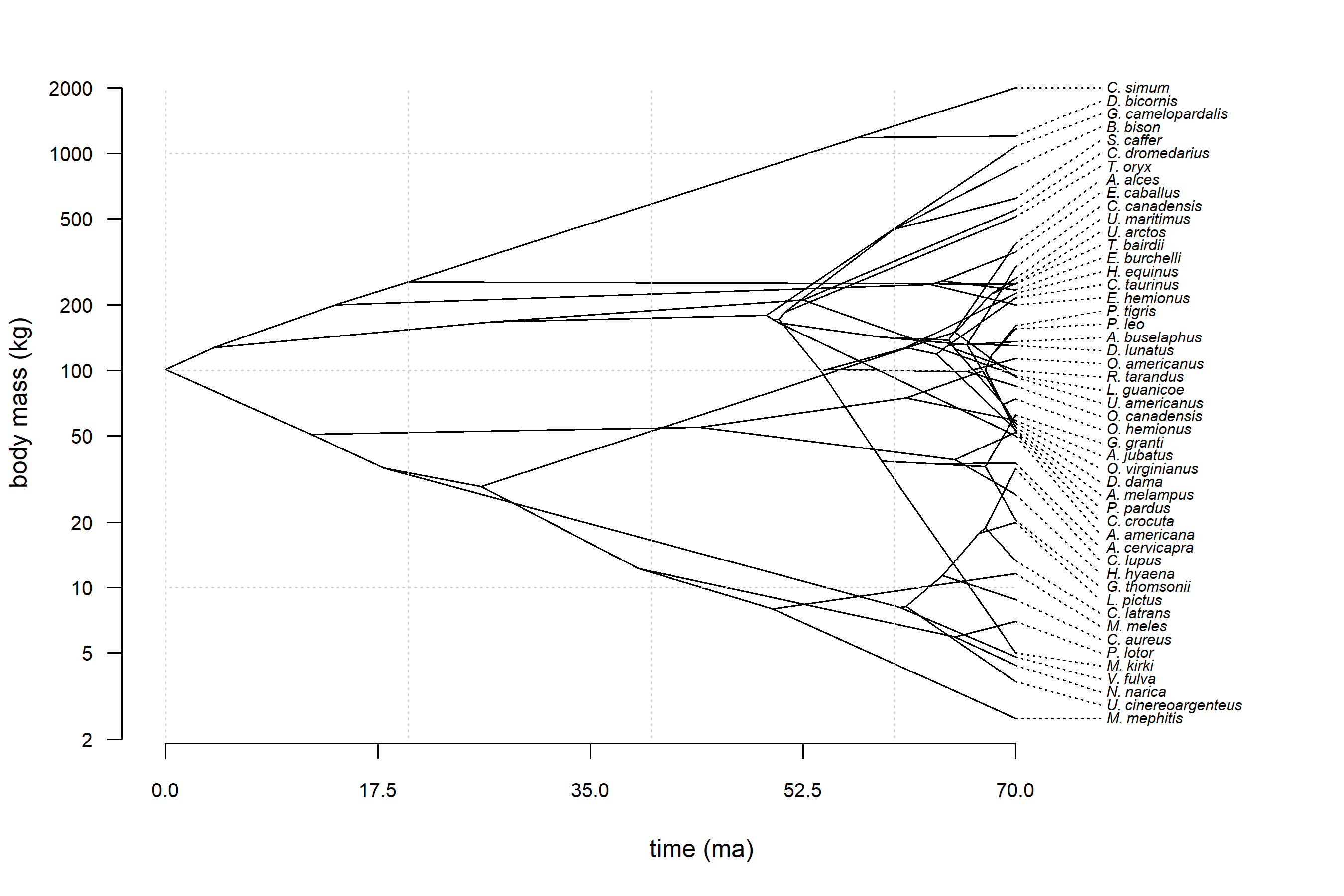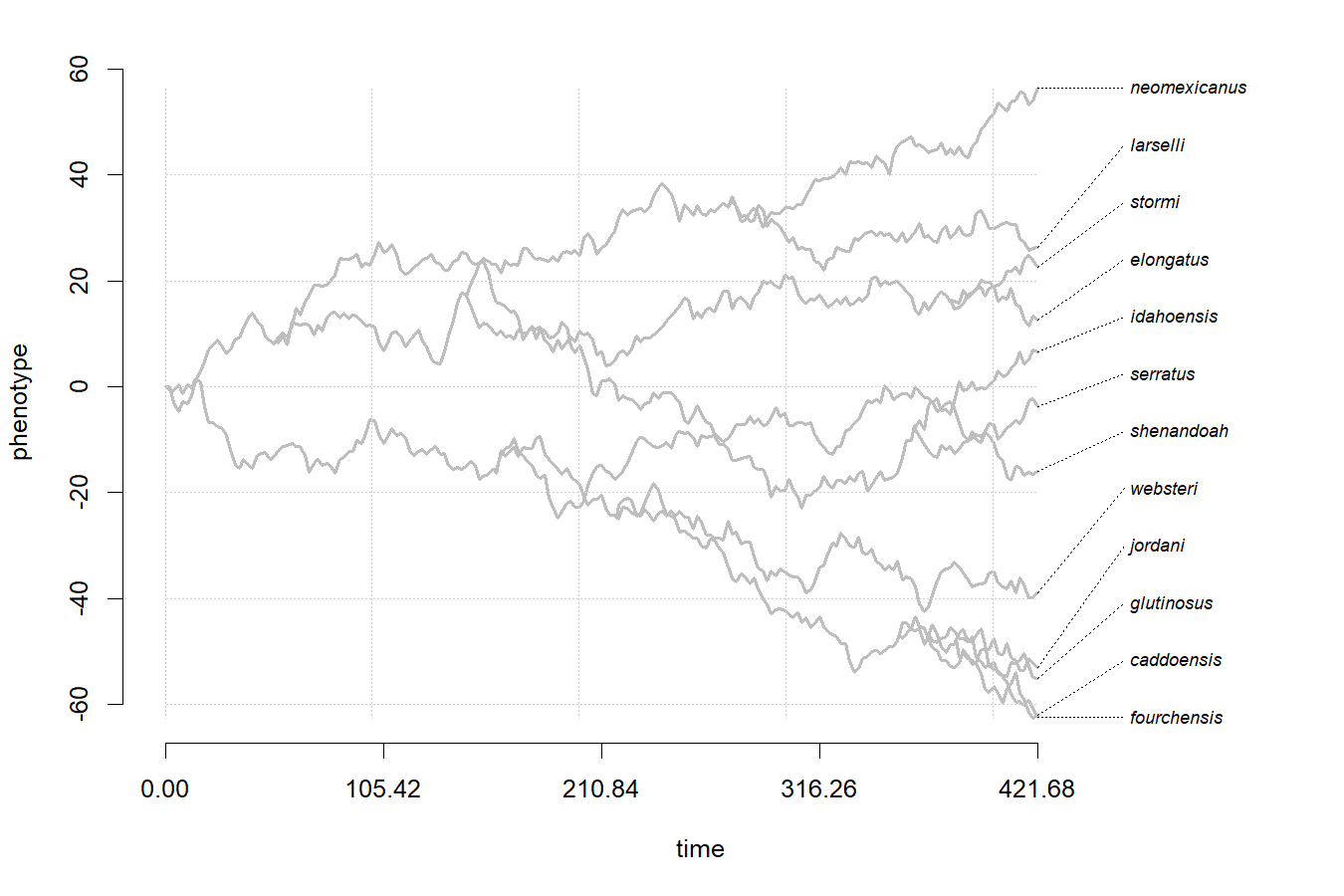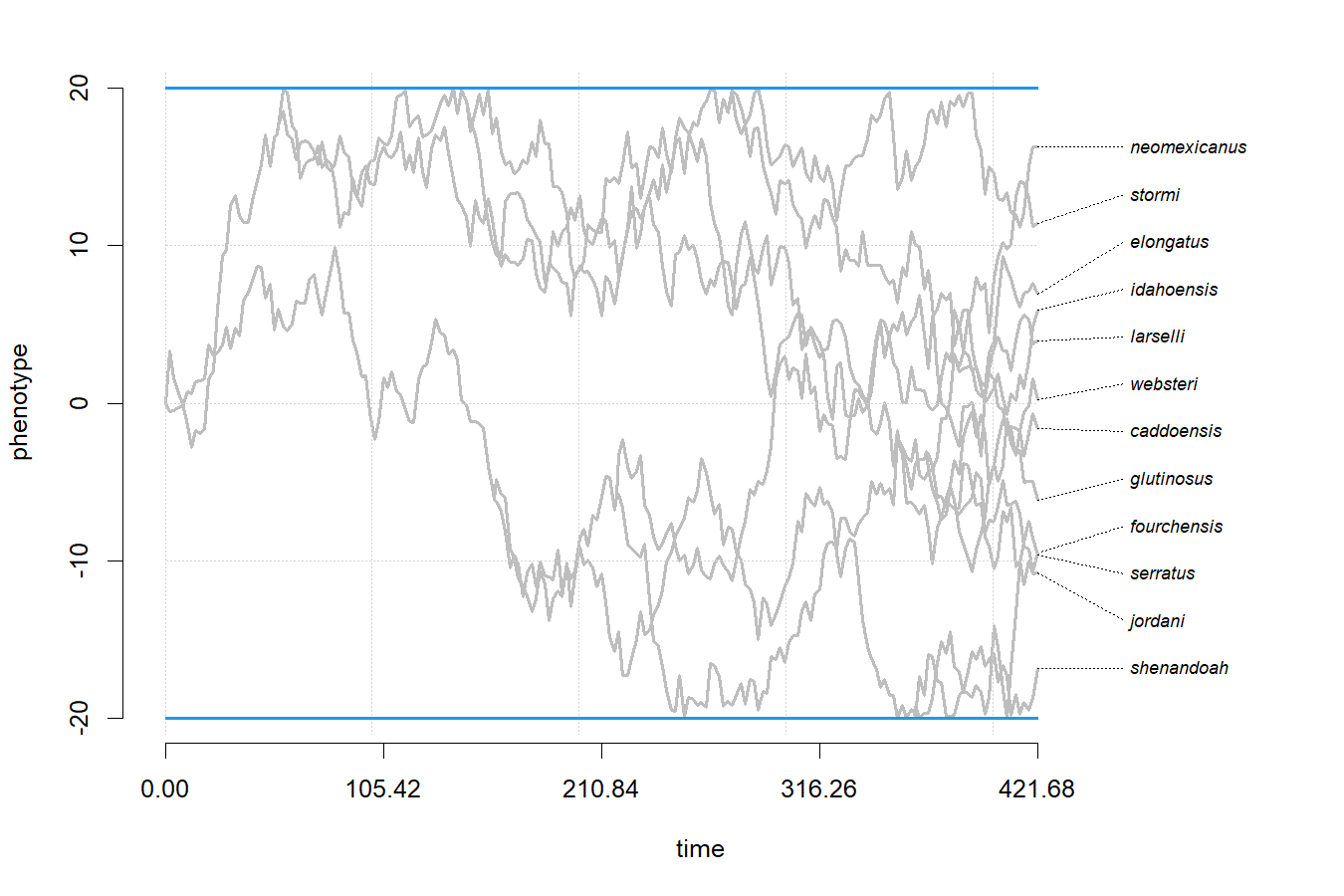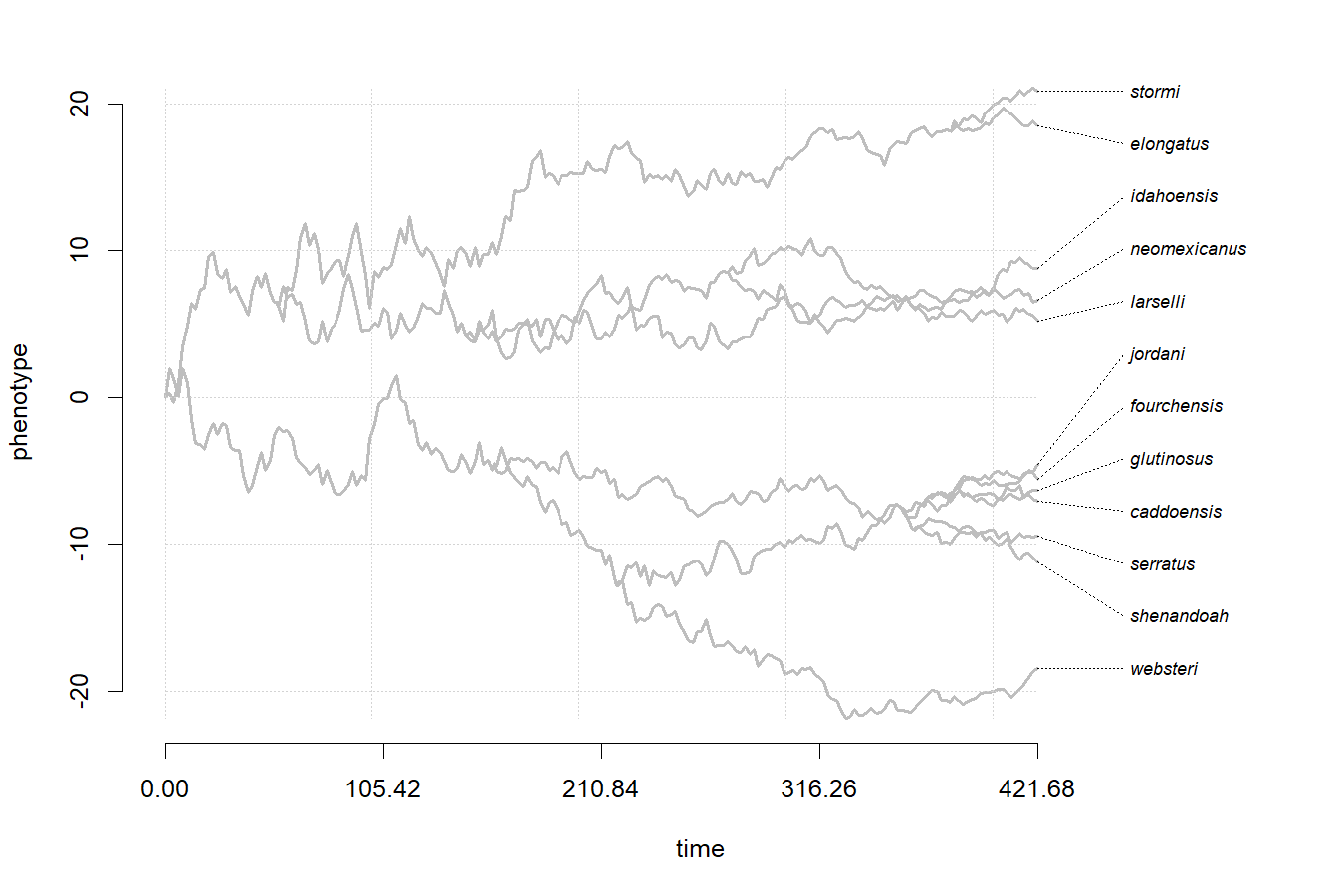## Thursday, November 25, 2021

### Plotting a phylogenetic traitgram to a PNG graphical device, and other tricks

A phytools user today (yes, Thanksgiving!) asked the following:

“When I use the phenogram() function to plot a traitgram in the RStudio plot window, what is the best way for me to then save that traitgram to my computer as an image? R doesn't really seem to understand that it's a plot object: ggsave() doesn't work, and when I assign the phenogram code to a variable, the object is only saving the xy coordinates of each node, not the topology or graphical options. Thus, using png() just produces a dotplot of those coordinates.”

The question is an interesting one, reflecting a difference between how a function like ggsave (which renders the graph from our current plot into a new device) and png (which creates a new device for us to graph to) work.

To use png (or, for example, pdf) we need to first open our png graphical device (using png), then run the code that creates our plot, then close our open graphical device using dev.off.

(Base R graphics also features functions that are more similar to the way I understand ggsave works. dev.copy2pdf is one example.)

To respond, I sent her the very simple partial example of:

png(file="Filename.png",width=7,height=7,units="in",res=300)
phenogram(...) ## your plotting code here
dev.off()


I wanted to give a more complete worked example; however, when I tried, I inadvertently discovered a couple of issues with the corresponding phytools functions or data!

First, I discovered that the dataset mammal.data (which is from Garland et al. 1992) had a error – the mass of S. caffer (the African buffalo) was accidentally recorded to be 10 $$\times$$ larger than it should have been!! (I don't know where the error came from, but the numbers are correct in Garland et al., as far as I can tell – so not there.)

Second, I also discovered that phenogram(...,log="y") doesn't quite work properly. The traitgram plots – but then the tip labels are not plotted, and (if they were) they would be spaced improperly!

I decided that these two issues would be easy to address, so I fixed them both, and now the updates are on the phytools GitHub.

Let's see the example I wanted to send!

library(phytools)
packageVersion("phytools")

##  '0.7.93'

data(mammal.tree)
data(mammal.data)
lnBodyMass<-setNames(log(mammal.data$bodyMass),rownames(mammal.data)) anc.lnBodyMass<-fastAnc(mammal.tree,lnBodyMass) bodyMass<-exp(c(anc.lnBodyMass,lnBodyMass)) png(file="Mammal-Traitgram.png",width=9,height=6,units="in",res=300) par(mar=c(5.1,4.1,2.1,2.1)) phenogram(mammal.tree,bodyMass,spread.cost=c(1,0),ftype="i",fsize=0.6, ylab="body mass (kg)",xlab="time (ma)",las=1,log="y",lwd=1, cex.axis=0.8)  ## Optimizing the positions of the tip labels...  clip(0,70,2,2000) ## clip plotting area grid() ## add grid dev.off()  ## png ## 2  The resultant plot is as follows:Working with phenogram I was also reminded of another neat trick that you can do with the function, and that is to use it to visualize simulated continuous trait evolution through time – including between nodes! The way to do that is to add a whole bunch of unbranching nodes along each edge of the original tree. I do this first using map.era.map (to create a whole bunch of 'regimes' on the tree) and then map.to.singleton (to convert these regimes into singleton or unbranching nodes). First, let's see standard Brownian motion evolution on a 12 taxon tree. I'll use a real tree of salamanders, but (remember) these are simulated data of course! data(salamanders) tree<-drop.tip(salamanders,sample(salamanders$tip.label,14))
h<-max(nodeHeights(tree))
tree<-make.era.map(tree,seq(0,0.995*h,by=0.005*h))
tree<-map.to.singleton(tree)
x<-fastBM(tree,internal=TRUE)
par(mar=c(5.1,4.1,2.1,2.1))
fsize=0.7)
clip(0,h,min(x),max(x))
grid()Cool. Now, let's try again – but this time, for bounded Brownian evolution!

tree<-make.era.map(collapse.singles(tree),
seq(0,0.995*h,by=0.005*h))
tree<-map.to.singleton(tree)
x<-fastBM(tree,internal=TRUE,bounds=c(-20,20))
par(mar=c(5.1,4.1,2.1,2.1))
fsize=0.7)
clip(0,h,-21,21)
grid()
abline(h=-20,col=palette(),lwd=2)
abline(h=20,col=palette(),lwd=2)Awesome. That's exactly what I was going for. Lastly, let's try an early-burst (“EB”) model.

tree<-make.era.map(collapse.singles(tree),
seq(0,0.995*h,by=0.005*h))
tree<-map.to.singleton(tree)
x<-fastBM(phytools:::ebTree(tree,-0.008),internal=TRUE)
par(mar=c(5.1,4.1,2.1,2.1))
fsize=0.7)
clip(0,h,min(x),max(x))
grid()That's it!